/

### Blending Independent Components and Principal Components Analysis 2.6 Infomax and maximum likelihood independent components analysis

Next page

2.6          Infomax and maximum likelihood independent components analysis

ICA as normally understood can be thought of as a multivariate, parallel version of projection pursuit, i.e. an algorithm that returns ‘all at once’ all of the unmixing weights applicable to all of the input signals. Indeed, if ICA uses the same measure of ‘signal-likeness’ (i.e. ‘independence’, ‘non-normality’, ‘lack of complexity’) and assumes the same number of signals exist as is used in the corresponding projection pursuit methodology then the two should extract the same signals.

To the extent that the two differ, the core measure of ‘signal-likeness’ underlying most implementations of ICA is that of statistical independence. As we have noted earlier, this is a stronger concept than mere lack of correlation. To make use of this idea, we need a measure that tells us how close to independent are any given set of unmixed signals.

Perhaps the most common measure used for this purpose is entropy. This is often thought of as a measure of the uniformity of the distribution of a bounded set of values. However, more generally, it can also be thought of as the amount of ‘surprise’ associated with a given outcome. This requires some a priori view of what probability distribution of outcomes is to be ‘expected’. Surprise can then equated with relative entropy (i.e. Kullback-Leibler divergence) which measures the similarity between two different probability density functions.

The ICA approach thus requires an assumed probability density function for the input signals and identifies the unmixing matrix that maximises the joint entropy of the resulting unmixed signals. This is called the infomax ICA approach. A common assumed probability density function (‘pdf’) used for this purpose is a very high-kurtosis one such as some suitably scaled version of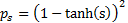.

ICA can also be thought of as a maximum likelihood method for estimating the optimal unmixing matrix. With maximum likelihood we again need to specify an a priori probability distribution, in this case the assumed joint pdf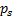of the unknown source signals, and we seek the unmixing matrix,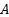, that yields extracted signals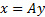with a joint pdf as similar as possible to. In such contexts, ‘as similar as possible’ is usually defined via the log likelihood function, which results in the same answer as the equivalent infomax approach, since both involve logarithmic functions of the underlying assumed probability distribution.

Both methods appear to rely on the frankly unrealistic assumption that the model pdf is an exact match for the pdf of the source signals. Of course, in general, the pdf of the source signals is not known exactly. Despite this, ICA seems to work reasonably well. This is because we do not really care about the form of the pdf. Indeed, it could correspond to a quite extreme distribution. Instead all we really need for the approach to work is for the model pdf to have the property that the closer any given distribution is to it (in relative entropy or log likelihood terms), the more likely that distribution is to correspond to a true source input signal. A hyperbolic tangent (‘tanh’)-style pdf may be an unrealistic ‘model’ for a true signal source, but use within the algorithm typically means that distributional forms with high kurtosis will be preferentially selected versus ones with lower kurtosis (even though neither may have a kurtosis anywhere near as large as that exhibited by the hyperbolic tangent pdf itself). It is the relative ordering of distributional forms introduced by choice of model pdf that is important rather than the structure of the model pdf per se. As a tanh-style model pdf preferentially extracts signals exhibiting high kurtosis it will extract similar signals to those extracted by kurtosis-based projection pursuit methods. Indeed, it ought to be possible to select model pdfs (or at least definitions of how to order likenesses of distributional forms to the model pdfs) that exactly match whatever metric is used in a corresponding projection pursuit methodology (even if this isn’t how the ICA methodology was originally developed).

To estimate the unmixing matrix(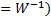that maximises the relative entropy or log likelihood and hence corresponds to the supposed input signals, we could again use brute force. However, again it is more efficient to use some sort of gradient ascent method as per Section 2.8, iteratively adjusting the estimated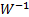in order to maximise the chosen metric.